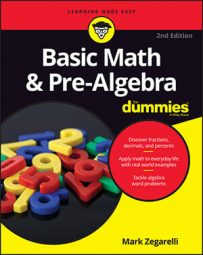##### Basic Math & Pre-Algebra For DummiesSome expressions contain only multiplication and division. When this is the case, the rule for evaluating the expression is pretty straightforward. When an expression contains only multiplication and division, evaluate it step by step from left to right.

## The Three Types of Big Four Expressions

Expression Example Rule
Contains only addition and subtraction 12 + 7 – 6 – 3 + 8 Evaluate left to right.
Contains only multiplication and division 18 ÷ 3 x 7 ÷ 14 Evaluate left to right.
Mixed-operator expression: contains a combination of addition/subtraction and multiplication/division 9 + 6 ÷ 3 1. Evaluate multiplication and division left to right. 2. Evaluate addition and subtraction left to right.

Suppose you want to evaluate this expression:

9 × 2 ÷ 6 ÷ 3 × 2
Again, the expression contains only multiplication and division, so you can move from left to right, starting with 9 x 2:
= 18 ÷ 6 ÷ 3 × 2 = 3 ÷ 3 × 2 = 1 × 2 = 2
Notice that the expression shrinks one number at a time until all that’s left is 2. So
9 × 2 ÷ 6 ÷ 3 × 2 = 2
Here’s another quick example:
−2 × 6 ÷ −4
Even though this expression has some negative numbers, the only operations it contains are multiplication and division. So you can evaluate it in two steps from left to right (remembering the rules for multiplying and dividing with negative numbers):
= −2 × 6 ÷ −4 = −12 ÷ −4 = 3
Thus,
−2 × 6 ÷ −4 = 3